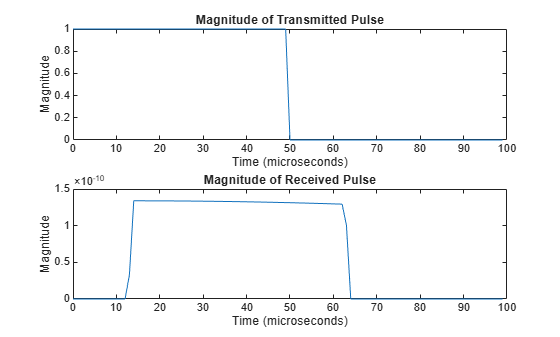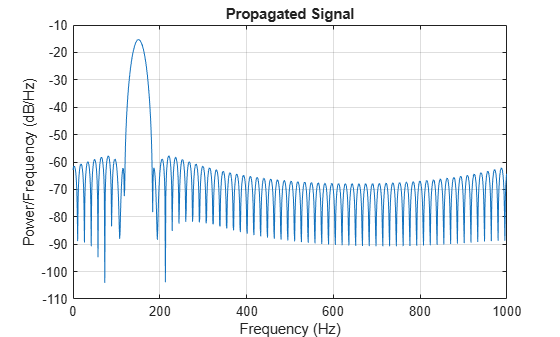## Free Space Path Loss

### Support for Modeling Propagation in Free Space

Propagation environments have significant effects on the amplitude, phase, and shape of propagating space-time wavefields. In some cases, you may want to simulate a system that propagates narrowband signals through free space. If so, you can use the `phased.FreeSpace` System object™ to model the range-dependent time delay, phase shift, Doppler shift, and gain effects.

Consider this object as a point-to-point propagation channel. By setting object properties, you can customize certain characteristics of the environment and the signals propagating through it, including:

• Propagation speed and sampling rate of the signal you are propagating

• Signal carrier frequency

• Whether the object models one-way or two-way propagation

Each time you call `step` on a `phased.FreeSpace` object, you specify not only the signal to propagate, but also the location and velocity of the signal origin and destination.

You can use `fspl` to determine the free space path loss, in decibels, for a given distance and wavelength.

### Free Space Path Loss in dB

Assume a transmitter is located at (1000,250,10) in the global coordinate system. Assume a target is located at (3000,750,20). The transmitter operates at 1 GHz. Determine the free space path loss in decibels for a narrowband signal propagating to and from the target.

`[tgtrng,~] = rangeangle([3000; 750; 20],[1000; 250; 10]);`

Multiply the range by two for two-way propagation.

`tgtrng = 2*tgtrng;`

Determine the wavelength for 1 GHz.

`lambda = physconst('LightSpeed')/1e9;`

Solve for the loss using `fspl`.

`L = fspl(tgtrng,lambda)`
```L = 104.7524 ```

The free space path loss in decibels is approximately 105 dB.

Alternatively, you can compute the loss directly from

`Loss = pow2db((4*pi*tgtrng/lambda)^2)`
```Loss = 104.7524 ```

### Propagate Linear FM Pulse Waveform to Target and Back

Construct a linear FM pulse waveform of 50 ms duration with a bandwidth of 100 kHz. Model the range-dependent time delay and amplitude loss incurred during two-way propagation. The pulse propagates between the transmitter located at (1000,250,10) and a target located at (3000,750,20). The signals propagate at the speed of light.

Note: This example runs only in R2016b or later. If you are using an earlier release, replace each call to the function with the equivalent `step` syntax. For example, replace `myObject(x)` with `step(myObject,x)`.

```waveform = phased.LinearFMWaveform('SweepBandwidth',1e5,... 'PulseWidth',5e-5,'OutputFormat','Pulses',... 'NumPulses',1,'SampleRate',1e6,'PRF',1e4); signal = waveform(); channel = phased.FreeSpace('SampleRate',1e6,... 'TwoWayPropagation',true,'OperatingFrequency',1e9); y = channel(signal,[1000; 250; 10],[3000; 750; 20],[0;0;0],[0;0;0]);```

Plot the magnitude of the transmitted and received pulse to show the amplitude loss and time delay.

```t = unigrid(0,1/waveform.SampleRate,1/waveform.PRF,'[)'); subplot(2,1,1) plot(t.*1e6,abs(signal)) title('Magnitude of Transmitted Pulse') xlabel('Time (microseconds)') ylabel('Magnitude') subplot(2,1,2) plot(t.*1e6,abs(y)) title('Magnitude of Received Pulse') xlabel('Time (microseconds)') ylabel('Magnitude')```The delay in the received pulse is approximately 14 μs, the expected value for a distance of 4.123 km.

### One-Way and Two-Way Propagation

The `TwoWayPropagation` property of the `phased.FreeSpace` System object™ lets you simulate either one- or two-way propagation. The following example demonstrates how to use this property for a single linear FM pulse propagating to a target and back. The sensor is a single isotropic radiating antenna operating at 1 GHz located at (1000,250,10). The target is located at (3000,750,20) and has a nonfluctuating RCS of 1 square meter.

Note: This example runs only in R2016b or later. If you are using an earlier release, replace each call to the function with the equivalent `step` syntax. For example, replace `myObject(x)` with `step(myObject,x)`.

The following code constructs the required System objects and calculates the range and angle from the antenna to the target.

```waveform = phased.LinearFMWaveform('SweepBandwidth',1e5,... 'PulseWidth',5e-5,'OutputFormat','Pulses',... 'NumPulses',1,'SampleRate',1e6); antenna = phased.IsotropicAntennaElement('FrequencyRange',[500e6 1.5e9]); transmitter = phased.Transmitter('PeakPower',1e3,'Gain',20); radiator = phased.Radiator('Sensor',antenna,'OperatingFrequency',1e9); channel = phased.FreeSpace('SampleRate',1e6,... 'TwoWayPropagation',true,'OperatingFrequency',1e9); target = phased.RadarTarget('MeanRCS',1,'Model','Nonfluctuating'); collector = phased.Collector('Sensor',antenna,'OperatingFrequency',1e9); sensorpos = [3000;750;20]; tgtpos = [1000;250;10]; [tgtrng,tgtang] = rangeangle(sensorpos,tgtpos);```

Because the `TwoWayPropagation` property is set to `true`, you compute the total propagation only once.

```pulse = waveform(); pulse = transmitter(pulse); pulse = radiator(pulse,tgtang);```

Propagate the pulse to the target and back.

```pulse = channel(pulse,sensorpos,tgtpos,[0;0;0],[0;0;0]); pulse = target(pulse); sig = collector(pulse,tgtang);```

Alternatively, you can break up the two-way propagation into two separate one-way propagation paths. You do so by setting the `TwoWayPropagation` property to `false`.

```channel1 = phased.FreeSpace('SampleRate',1e9,... 'TwoWayPropagation',false,'OperatingFrequency',1e6);```

```pulse = waveform(); pulse = transmitter(pulse); pulse = radiator(pulse,tgtang);```

Propagate the pulse from the antenna to the target.

```pulse = channel1(pulse,sensorpos,tgtpos,[0;0;0],[0;0;0]); pulse = target(pulse);```

Propagate the reflected pulse from the target to the antenna.

```pulse = channel(pulse,tgtpos,sensorpos,[0;0;0],[0;0;0]); sig = collector(pulse,tgtang);```

### Propagate Signal from Stationary Radar to Moving Target

This example shows how to propagate a signal in free space from a stationary radar to a moving target.

Note: This example runs only in R2016b or later. If you are using an earlier release, replace each call to the function with the equivalent `step` syntax. For example, replace `myObject(x)` with `step(myObject,x)`.

Define the signal sample rate, propagation speed, and carrier frequency. Define the signal as a sinusoid of frequency 150 Hz. Set the sample rate to 1 kHz and the carrier frequency to 300 MHz. The propagation speed is the speed of light.

```fs = 1.0e3; c = physconst('Lightspeed'); fc = 300e3; f = 150.0; N = 1024; t = (0:N-1)'/fs; x = exp(1i*2*pi*f*t);```

Assume the target is approaching the radar at 300.0 m/s, and the radar is stationary. Find the Doppler shift that corresponds to this relative speed.

```v = 1000.0; dop = speed2dop(v,c/fc)```
```dop = 1.0007 ```

From the formula, the one-way doppler shift is 1 Hz.

Create a `phased.FreeSpace` System object™, and use it to propagate the signal from the radar to the target. Assume the radar is at (0, 0, 0) and the target is at (100, 0, 0).

```channel = phased.FreeSpace('SampleRate',fs,... 'PropagationSpeed',c,'OperatingFrequency',fc); origin_pos = [0;0;0]; dest_pos = [100;0;0]; origin_vel = [0;0;0]; dest_vel = [-v;0;0]; y = channel(x,origin_pos,dest_pos,origin_vel,dest_vel);```

Plot the spectrum of the transmitted signal. The peak at 150 Hz reflects the frequency of the signal.

```window = 64; ovlp = 32; [Pxx,F] = pwelch(x,window,ovlp,N,fs); plot(F,10*log10(Pxx)) xlabel('Frequency (Hz)') ylabel('Power/Frequency (dB/Hz)') title('Transmitted Signal')```Plot the spectrum of the propagated signal. The peak at 250 Hz reflects the frequency of the signal plus the Doppler shift of 100 Hz.

```window = 64; ovlp = 32; [Pyy,F] = pwelch(y,window,ovlp,N,fs); plot(F,10*log10(Pyy)) grid xlabel('Frequency (Hz)') ylabel('Power/Frequency (dB/Hz)') title('Propagated Signal')```The doppler shift is too small to see. Overlay the two spectra in the region of 150 Hz.

```figure idx = find(F>=130 & F<=170); plot(F(idx),10*log10(Pxx(idx)),'b') grid hold on plot(F(idx),10*log10(Pyy(idx)),'r') hold off xlabel('Frequency (Hz)') ylabel('Power/Frequency (dB/Hz)') title('Transmitted and Propagated Signals') legend('Transmitted','Propagated')```The peak shift appears to be approximately 1 Hz.